• 今天小编就为大家分享一篇python 和c++实现旋转矩阵欧拉角的变换方式，具有很好的参考价值，希望对大家有所帮助。一起跟随小编过来看看吧
• #ifndef IMGPOSTCC_HPP #define IMGPOSTCC_HPP #include "Eigen/Dense" class imgpostCC { // public: void Demo(){ //using namespace Eigen;... double pin, rin, yin, pout, rout, yout;... .
#ifndef IMGPOSTCC_HPP
#define IMGPOSTCC_HPP

#include "Eigen/Dense"

class imgpostCC {
//
public:
void Demo(){
//using namespace Eigen;

double pin, rin, yin, pout, rout, yout;
//初始化
setCameraCaliPara(-90, 0, 0);

pin = 0;
rin = 45;
yin = 0;
//计算
getRotationENUtoIMU(pin, rin, yin, pout, rout, yout);

#if 0
//zxy
Eigen::Vector3d v = printEulerAngles(imu_pry_R,2,0,1);
printf("imu_pry_R: x:%.2f y:%.2f z:%.2f\r\n",
v * 180.0 / M_PI,
v * 180.0 / M_PI,
v * 180.0 / M_PI);

//zyx
v = printEulerAngles(cam_cali_R, 2, 1, 0);
printf("cam_cali_R: x:%.2f y:%.2f z:%.2f\r\n",
v * 180.0 / M_PI,
v * 180.0 / M_PI,
v * 180.0 / M_PI);

//zxy
v = printEulerAngles(enu_cam_R,2,0,1);
printf("enu_cam_R: pitch:%.2f roll:%.2f yaw:%.2f\r\n",
v * 180.0 / M_PI,
v * 180.0 / M_PI,
v * 180.0 / M_PI);
#endif

}

void setCameraCaliPara(double rx, double ry, double rz){
Eigen::AngleAxisd rx_cam,ry_cam,rz_cam;
rx_cam = Eigen::AngleAxisd(rx / 180.0 * M_PI, Eigen::Vector3d::UnitX());
ry_cam = Eigen::AngleAxisd(ry / 180.0 * M_PI, Eigen::Vector3d::UnitY());
rz_cam = Eigen::AngleAxisd(rz / 180.0 * M_PI, Eigen::Vector3d::UnitZ());
//z - y - x
cam_cali_R = Eigen::AngleAxisd(rz_cam * ry_cam * rx_cam);
}

void getRotationENUtoIMU(double pitch, double roll, double yaw, double& p, double& r, double& y){
//zxy
Eigen::AngleAxisd rx_imu,ry_imu,rz_imu;
rx_imu = Eigen::AngleAxisd(pitch / 180.0 * M_PI, Eigen::Vector3d::UnitX());
ry_imu = Eigen::AngleAxisd(roll / 180.0 * M_PI, Eigen::Vector3d::UnitY());
//西偏为正
rz_imu = Eigen::AngleAxisd(-yaw / 180.0 * M_PI, Eigen::Vector3d::UnitZ());
//z - x - y
imu_pry_R = Eigen::AngleAxisd(rz_imu * rx_imu * ry_imu);
//R(enu2camera)=R(enu2imu)*R(imu2camera)
enu_cam_R = Eigen::AngleAxisd(imu_pry_R * cam_cali_R);
//enu2camera - z - x - y
Eigen::Vector3d eulerAngle = enu_cam_R.matrix().eulerAngles(2,0,1);

p = eulerAngle * 180.0 / M_PI;
r = eulerAngle * 180.0 / M_PI;
y = eulerAngle * 180.0 / M_PI;
printf("enu_cam_R: pitch:%.2f roll:%.2f yaw:%.2f\r\n", p, r, y);
}

private:

Eigen::Vector3d printEulerAngles(Eigen::AngleAxisd aa, int id0, int id1, int id2){
//zxy
Eigen::Vector3d eulerAngle = aa.matrix().eulerAngles(id0,id1,id2);
return eulerAngle;
}

public:
Eigen::AngleAxisd cam_cali_R, imu_pry_R, enu_cam_R;
};

#endif // IMGPOSTCC_HPP

展开全文• 旋转矩阵转欧拉角概述欧拉角旋转矩阵欧拉角转为旋转矩阵旋转矩阵转为欧拉角总结 概述 本篇博客，主要给出如何将3x3的旋转矩阵转换成欧拉角的讨论和代码。 什么是旋转矩阵欧拉角呢？ 欧拉角 我们应该知道，对于...

概述

本篇博客，主要给出如何将3x3的旋转矩阵转换成欧拉角的讨论和代码。
什么是旋转矩阵和欧拉角呢？

欧拉角

我们应该知道，对于一个三维空间，我们可以建立一个三维坐标轴，而这个三维坐标轴由X轴，Y轴和Z轴组成。当三维物体进行旋转时，可以看成该物体依次绕着这三个轴进行某种角度的旋转，这些角度被称为欧拉角。值得注意的是，在行业中，欧拉角饶轴旋转的循序是Z-Y-X，因为这样对应着yaw-pitch-roll。

yaw：偏航角，指物体绕着Z轴旋转
pitch：俯仰角，指物体绕着Y轴旋转
roll：滚转角，指物体绕着X轴旋转

欧拉角看起来比较简单，但是它的一个重大缺点是会出现万向锁问题：在俯仰角为 ± 9 0 ° \pm90^° 时，第一次旋转与第三次旋转将使用同一个轴，这使得系统丢失了一个自由度。

旋转矩阵

旋转矩阵的几何意义也很明确，把一个三维空间点(x,y,z)看为一个三维向量[x,y,z]，然后将该向量与一个矩阵相乘，得到一个变换后的三维向量。值得注意的一点是，如果你将三维空间点看成一个行向量，那旋转矩阵是在乘号后边，即VM。但是如果使用列向量表示，那旋转矩阵是在乘号前边，即MV。而这两种情况下的旋转矩阵M是不相同的(他们是彼此的转置)

将欧拉角转为旋转矩阵

考虑三维旋转最简单的方式是轴角形式。因为任何旋转都可以由旋转轴和描述旋转量的角度来定义。
由上述的讨论可知，每个轴都有其对应的旋转矩阵。下面分别给出X轴，Y轴和Z轴的旋转矩阵。其中， θ x , θ y , θ z \theta_x,\theta_y,\theta_z 对应为每个轴的旋转角度，即欧拉角。
为了得到最后的旋转矩阵 R R ，我们需要依次将物体在这些矩阵上进行连续运算，因此，最后的 R R 可以表示为如下的矩阵乘法。从欧拉角转换为旋转矩阵的函数片段如下：

def eulerAnglesToRotationMatrix(theta) :
# 分别构建三个轴对应的旋转矩阵
R_x = np.array([[1,         0,                  0                   ],
[0,         math.cos(theta), -math.sin(theta) ],
[0,         math.sin(theta), math.cos(theta)  ]
])

R_y = np.array([[math.cos(theta),    0,      math.sin(theta)  ],
[0,                     1,      0                   ],
[-math.sin(theta),   0,      math.cos(theta)  ]
])

R_z = np.array([[math.cos(theta),    -math.sin(theta),    0],
[math.sin(theta),    math.cos(theta),     0],
[0,                     0,                      1]
])

# 将三个矩阵相乘，得到最终的旋转矩阵
R = np.dot(R_z, np.dot( R_y, R_x ))

return R

将旋转矩阵转为欧拉角

将旋转矩阵转换为欧拉角有点困难，因为在大多数情况下，解决方案不是唯一的。下面代码的输出完全与Matlabrotm2euler函数一致。

首先，先对旋转矩阵进行检查

# 检查一个旋转矩阵是否有效
def isRotationMatrix(R) :
# 得到该矩阵的转置
Rt = np.transpose(R)
# 旋转矩阵的一个性质是，相乘后为单位阵
shouldBeIdentity = np.dot(Rt, R)
# 构建一个三维单位阵
I = np.identity(3, dtype = R.dtype)
# 将单位阵和旋转矩阵相乘后的值做差
n = np.linalg.norm(I - shouldBeIdentity)
# 如果小于一个极小值，则表示该矩阵为旋转矩阵
return n < 1e-6

完整的转换代码

# 这部分的代码输出与Matlab里边的rotm2euler一致
def rotationMatrixToEulerAngles(R) :
# 断言判断是否为有效的旋转矩阵
assert(isRotationMatrix(R))

sy = math.sqrt(R[0,0] * R[0,0] +  R[1,0] * R[1,0])

singular = sy < 1e-6

if  not singular :
x = math.atan2(R[2,1] , R[2,2])
y = math.atan2(-R[2,0], sy)
z = math.atan2(R[1,0], R[0,0])
else :
x = math.atan2(-R[1,2], R[1,1])
y = math.atan2(-R[2,0], sy)
z = 0

return np.array([z, y, x])

总结

三维旋转是一个十分常见的需求，这些代码都可以直接使用，非常的nice。如果对完整代码有需求的小伙伴，可以去原网站下载，嫌麻烦，也可以下载我添加上注释后的版本

展开全文线性代数 matlab python 计算机视觉
• 旋转矩阵计算绕X、Y、Z轴旋转角度的计算公式，直接代入公式求解即可，适用于3*3旋转矩阵的计算。
• 在机器人运动中经常涉及到坐标变换，而根据旋转矩阵欧拉角需要考虑坐标轴的旋转顺序，文档中列出了不同选择顺序对应的旋转矩阵以及相应的求解欧拉角公式旋转矩阵 欧拉角
• 今天小编就为大家分享一篇Python根据欧拉角旋转矩阵的实例，具有很好的参考价值，希望对大家有所帮助。一起跟随小编过来看看吧
• 旋转矩阵欧拉角， 用于有已知的旋转矩阵推算旋转角度。 对于任何形式的旋转矩阵有一定的参考价值。旋转矩阵 欧拉角
• Thisdocumentdiscussesasimpletechniquetoﬁ...本文档讨论了从旋转矩阵中提取可能的椭圆的完整技术。 确定欧拉角有时是计算机图形学，视觉，机器人和运动学中的必要步骤。 但是，解决方案可能会或可能不明显。旋转矩阵 欧拉角
• 这篇博客将会分享旋转矩阵欧拉角的相互转换。 三维旋转矩阵有三个自由度，旋转能够使用多种方法表示，比如一个3x3的矩阵，比如四元数，甚至可以将旋转表示为三个数字，即绕三个轴x,y,z的旋转角度。在原始的欧拉角...

这篇博客将会分享旋转矩阵和欧拉角的相互转换。

三维旋转矩阵有三个自由度，旋转能够使用多种方法表示（旋转矩阵，欧拉角，四元数，轴角，李群与李代数），比如一个3x3的矩阵，比如四元数，甚至可以将旋转表示为三个数字，即绕三个轴x,y,z的旋转角度。在原始的欧拉角公式中，旋转是通过围绕Z轴，Y轴，X轴分别旋转得到的。它分别对应于偏航，俯仰和横滚。

当定义一个旋转时，可能还会引起歧义，比如对于一个给定的点(x,y,z),可以把这个点视为行向量（x,y,z）或者列向量量 [ x , y , z ] T [x, y, z]^{T} ，如果使用行向量，那么就必须后乘旋转矩阵（旋转矩阵在后），如果使用列向量，就要左乘旋转矩阵（旋转矩阵在前）。

在MATLAB中，rotm2euler.m能够实现旋转矩阵到欧拉角的转换。下文中将要叙述的转换代码参考于MATLAB中的rotm2euler.m实现。不同于MATLAB实现的是，它的旋转顺序是Z-Y-X，而下面的实现是X-Y-Z。

在计算将旋转矩阵转换成欧拉角之前，先介绍一下欧拉角转换为旋转矩阵的方法。

欧拉角转换为旋转矩阵

假如已知旋转角，绕着X-Y-Z三个轴的角度分别为 θ x θ y θ z \theta_{x} \quad \theta_{y} \quad \theta_{z} 那么三个旋转矩阵可以表示如下：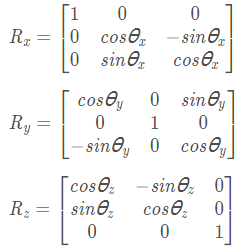如果旋转顺序为Z-Y-X的顺序，那么旋转矩阵可表示如下：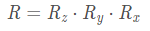关于上面的旋转矩阵，以z轴旋转为例：
第一种物理意义，坐标系的旋转。 其应用场景有SLAM，机械臂运动学等。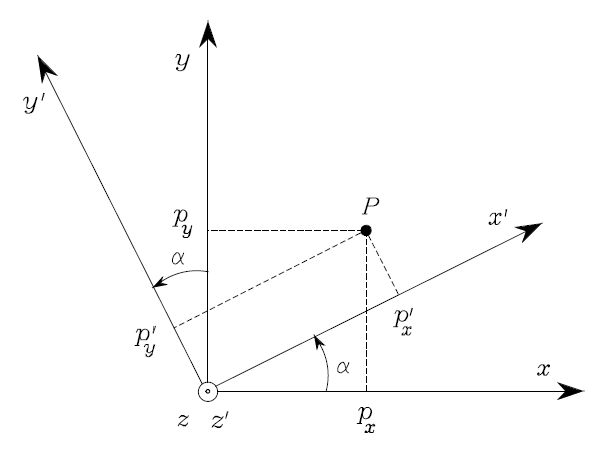如上图所示， P点不变，坐标系 O-XYZ旋转 α ,得到新的坐标系O-X’Y’Z’，在新坐标系下，P点的坐标变为P’ ，则有：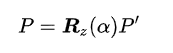第二种物理意义，向量的旋转，其应用场景有机器人的姿态估计等。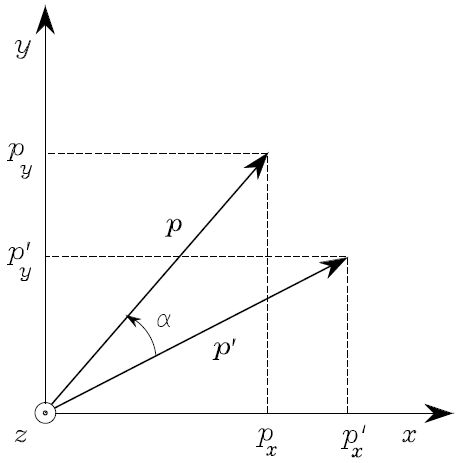如上图所示，坐标系 O-XYZ不变， P’点旋转α ,得到新的点P （我也想反过来，但用的教材上的图，没办法），则有：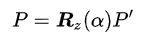对应于上述旋转顺序的C++代码如下：

C++
// Calculates rotation matrix given euler angles.
Mat eulerAnglesToRotationMatrix(Vec3f &theta)
{
// Calculate rotation about x axis
Mat R_x = (Mat_<double>(3,3) <<
1,       0,              0,
0,       cos(theta),   -sin(theta),
0,       sin(theta),   cos(theta)
);

// Calculate rotation about y axis
Mat R_y = (Mat_<double>(3,3) <<
cos(theta),    0,      sin(theta),
0,               1,      0,
-sin(theta),   0,      cos(theta)
);

// Calculate rotation about z axis
Mat R_z = (Mat_<double>(3,3) <<
cos(theta),    -sin(theta),      0,
sin(theta),    cos(theta),       0,
0,               0,                  1);

// Combined rotation matrix
Mat R = R_z * R_y * R_x;
return R;

}

Python
# Calculates Rotation Matrix given euler angles.
def eulerAnglesToRotationMatrix(theta) :

R_x = np.array([[1,                  0,                   0],
[0, math.cos(theta), -math.sin(theta)],
[0,  math.sin(theta), math.cos(theta) ]])

R_y = np.array([[math.cos(theta), 0, math.sin(theta)],
[0,                  1,                  0],
[-math.sin(theta), 0, math.cos(theta)]])

R_z = np.array([[math.cos(theta), -math.sin(theta), 0],
[math.sin(theta), math.cos(theta),  0],
[0,                  0,                   1]])

R = np.dot(R_z, np.dot( R_y, R_x ))

return R

在OpenCV中将旋转矩阵转换为欧拉角

将旋转矩阵转换为欧拉角就不是那么容易，不同的旋转顺序对应着不同的旋转角度。使用上面的代码，即使欧拉角看起来非常不同，您也可以验证与欧拉角[0.1920、2.3736、1.1170]（或[[11，136，64]度）和[-2.9496，0.7679，-2.0246]（或[-169，44，-116]度）相对应的旋转矩阵实际上是相同的。下面的代码显示了在给定旋转矩阵的情况下找到欧拉角的方法。以下代码的输出应与MATLAB的rotm2euler的输出完全匹配，但x和z的顺序会互换。

C++
// Checks if a matrix is a valid rotation matrix.
bool isRotationMatrix(Mat &R)
{
Mat Rt;
transpose(R, Rt);
Mat shouldBeIdentity = Rt * R;
Mat I = Mat::eye(3,3, shouldBeIdentity.type());

return  norm(I, shouldBeIdentity) < 1e-6;

}

// Calculates rotation matrix to euler angles
// The result is the same as MATLAB except the order
// of the euler angles ( x and z are swapped ).
Vec3f rotationMatrixToEulerAngles(Mat &R)
{

assert(isRotationMatrix(R));

float sy = sqrt(R.at<double>(0,0) * R.at<double>(0,0) +  R.at<double>(1,0) * R.at<double>(1,0) );

bool singular = sy < 1e-6; // If

float x, y, z;
if (!singular)
{
x = atan2(R.at<double>(2,1) , R.at<double>(2,2));
y = atan2(-R.at<double>(2,0), sy);
z = atan2(R.at<double>(1,0), R.at<double>(0,0));
}
else
{
x = atan2(-R.at<double>(1,2), R.at<double>(1,1));
y = atan2(-R.at<double>(2,0), sy);
z = 0;
}
return Vec3f(x, y, z);
}

Python
# Checks if a matrix is a valid rotation matrix.
def isRotationMatrix(R) :
Rt = np.transpose(R)
shouldBeIdentity = np.dot(Rt, R)
I = np.identity(3, dtype = R.dtype)
n = np.linalg.norm(I - shouldBeIdentity)
return n < 1e-6

# Calculates rotation matrix to euler angles
# The result is the same as MATLAB except the order
# of the euler angles ( x and z are swapped ).
def rotationMatrixToEulerAngles(R) :

assert(isRotationMatrix(R))

sy = math.sqrt(R[0,0] * R[0,0] +  R[1,0] * R[1,0])

singular = sy < 1e-6

if  not singular :
x = math.atan2(R[2,1] , R[2,2])
y = math.atan2(-R[2,0], sy)
z = math.atan2(R[1,0], R[0,0])
else :
x = math.atan2(-R[1,2], R[1,1])
y = math.atan2(-R[2,0], sy)
z = 0

return np.array([x, y, z])

展开全文旋转矩阵 欧拉角
• 旋转矩阵、欧拉角之间转换

千次阅读 2019-09-23 16:49:38
MATLAB矩阵乘法从左到右依次相乘 用R表示旋转矩阵。 yaw pitch roll分别表示Z　Ｙ　Ｘ轴的转角。 q=[q0,q1,q2,q3]'表示单位四元数。

学习过程中涉及欧拉角和旋转矩阵的转换，索性整理学习一下欧拉角四元数和旋转矩阵的概念以及matlab中的互相转换
本文摘自各大课本，博客，自己学习整理使用，侵删
MATLAB矩阵乘法从左到右依次相乘
用R表示旋转矩阵。
yaw（偏航） pitch（俯仰） roll（横滚）分别表示Z　Ｙ　Ｘ轴的转角。
q=[q0,q1,q2,q3]'表示单位四元数。

1旋转矩阵（方向余弦矩阵）

当确定一个点在空间中的位置后，要确定其姿态才能完全定义该点的位姿。所以采用坐标系{B}相对于坐标系{A}的描述来表示物体姿态，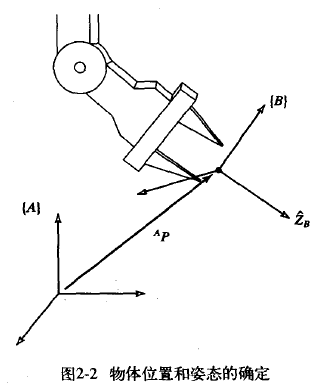通俗的讲，是坐标系{B}的各个轴分别与参考坐标系{A}的各个轴的余弦值构成的3x3矩阵，称为旋转矩阵。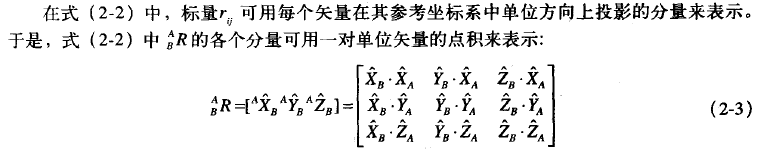旋转矩阵是一种特殊的各列相互正交的单位阵，旋转矩阵的行列式恒为1，旋转矩阵也称为标准正交矩阵。
旋转矩阵包含9个参数来描述一个姿态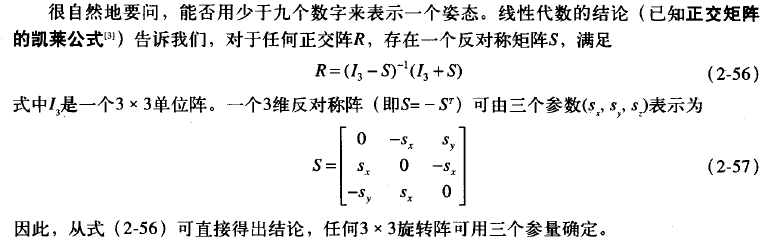欧拉角

1、内旋和外旋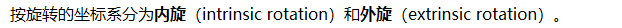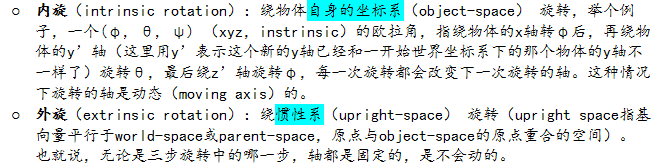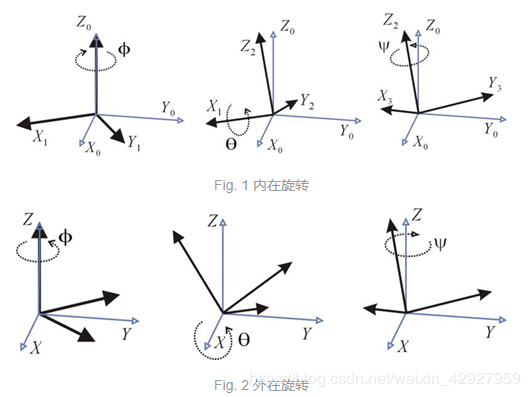内在旋转与外在旋转的转换关系：互换第一次和第三次旋转的位置则两者结果相同。例如Z-Y-X旋转的内部旋转和X-Y-Z旋转的外部旋转的旋转矩阵相同。

一、绕定轴X-Y-Z旋转（RPY角）（外旋）

假设两个坐标系A和B，二者初始时完全重合。

过程如下：B绕A的X轴旋转γ角，再绕A的Y轴旋转β角，最后绕A的Z轴旋转α角，完成旋转。整个过程，A不动B动。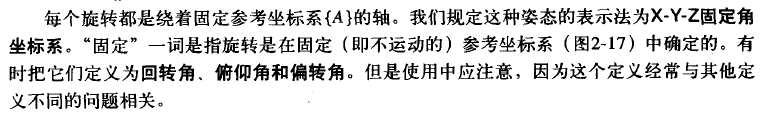旋转矩阵的计算方法如下：R = Rz * Ry *Rx，乘法顺序：从右向左，依次旋转X轴Y轴Z轴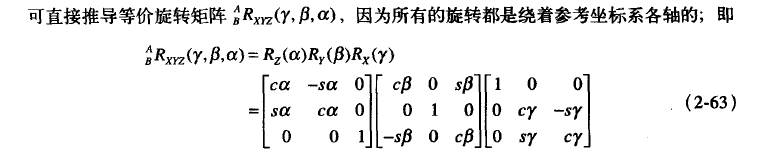其中，cα = cosα，sα = sinα，矩阵相乘，结果如下：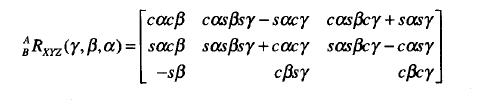二、绕动轴Z-Y-X旋转（Euler角）（内旋）

过程如下：B绕B的Z轴旋转α角，再绕B的Y轴旋转β角，最后绕B的X轴旋转γ角，完成旋转。整个过程，A不动B动。
旋转矩阵的计算方法如下：R = Rz * Ry *Rx。乘法顺序：从左向右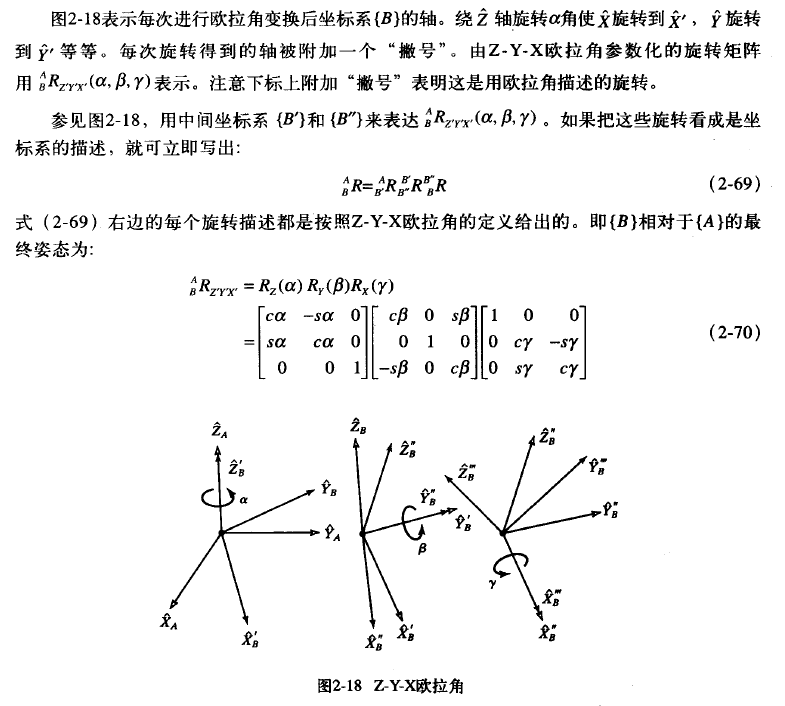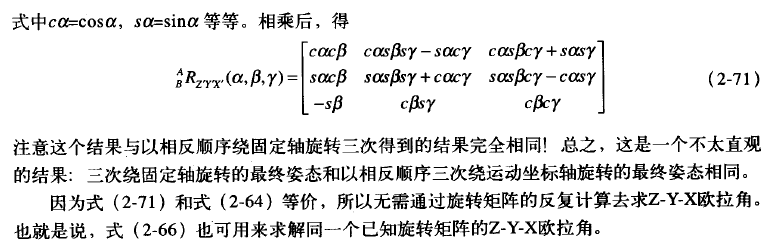欧拉角的表示方式比较直观，但是有几个缺点：

(1) 欧拉角的表示方式不唯一。给定某个起始朝向和目标朝向，即使给定yaw、pitch、roll的顺序，也可以通过不同的yaw/pitch/roll的角度组合来表示所需的旋转。比如，同样的yaw-pitch-roll顺序，(0,90,0)和(90,90,90)会将刚体转到相同的位置。这其实主要是由于万向锁(Gimbal Lock)引起的

(2) 欧拉角的插值比较难。

(3) 计算旋转变换时，一般需要转换成旋转矩阵，这时候需要计算很多sin, cos，计算量较大。

欧拉角转旋转矩阵

在计算坐标变换时，旋转更方便的表示形式是旋转矩阵(Rotation Matrix)。三维空间的旋转矩阵可以表示成3x3的矩阵，将欧拉角转换为旋转矩阵的计算方式如下，假设欧拉角yaw、pitch、roll的角度为alpha, beta, gamma，则旋转矩阵可以计算如下：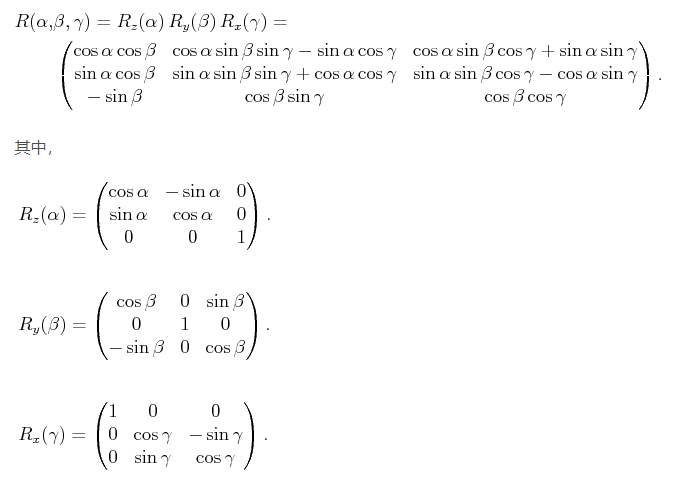eul2rotm(eul)中，默认旋转顺序是ZYX,而矩阵相乘顺序是从右到左，先X再Y后Z，
即：若eul(Z Y X)=eul(angle1 angle2 angle3)，则eul2rotm(eul,sequence)=Rot(Z,angle1)*Rot(Y,angle2)*Rot(X,angle3).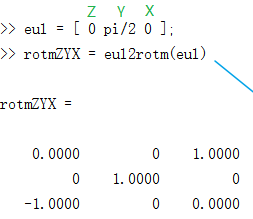rotm2eul()
计算单位为弧度制，计算结果的顺序对应欧拉角旋转轴顺序

%    Z   Y  X
eul=[0 pi/2 0];
yaw=0;
pitch=pi/2'
roll=0;
R_x=[1 0 0;
0 cos(roll) -sin(roll);
0 sin(roll) cos(roll)];
R_y=[cos(pitch) 0 sin(pitch);
0 1 0;
-sin(pitch) 0 cos(pitch)];
R_z=[cos(yaw) -sin(yaw) 0;
sin(yaw) cos(yaw) 0;
0 0 1];
R=R_z*R_y*R_x;%欧拉角转旋转矩阵
R2=angle2dcm(eul,'ZYX');%由欧拉角转方向余弦矩阵
R2=eul2rotm(eul,'ZYX');%欧拉角转旋转矩阵

四元数（后续补充）

一篇介绍四元数的文章
在Matlab里，可以用quatmultiply计算四元数乘法，用quatinv来计算四元数的逆，用quatconj来计算四元数的共轭。四元数的旋转和旋转矩阵的旋转可以由以下matlab代码验证：

% Matlab code by MulinB, Aerospace Toolbox is needed

pt = [10,20,30]; % point coordinate

yaw =   45;
pitch = 30;
roll =  60;

q = angle2quat(yaw/180*pi,pitch/180*pi,roll/180*pi);
R = angle2dcm(yaw/180*pi,pitch/180*pi,roll/180*pi);

pt1 = R*pt';
pt2 = quatmultiply(quatconj(q), quatmultiply([0,pt],q)); % NOTE the order
disp(pt1');disp(pt2(2:4));

从上述代码里也可以看到四元数和欧拉角和dcm的转换，在matlab里可以很方便的用quat, dcm, angle之间的转换来任意互转。另外，从四元数计算axis和angle，可以用以下代码计算：

% Matlab code by MulinB, Compute the axis and angle from a quaternion
function [axis, theta] = quat2axisangle(q)

theta = acos(q(1)) * 2;
axis = q(2:4)/sin(theta/2);

总结：

转欧拉角：

旋转矩阵转欧拉角
[r2,r2,r3]=dcm2angle(R, S)
eul=rotm2rul(R,S)

注：得到的结果为弧度，若需要角度需进一步转化
四元数转欧拉角
[r1,r2,r3]=quat2angle([q0 q1 q2 q3]，S)
注：S 的选择有12种，【‘ZYX’,‘ZYZ’,‘ZXY’,‘ZXZ’,‘YXZ’,‘YXY’,‘YZX’,‘YZY’,‘XYZ’,‘XYX’,‘XZY’,‘XZX’】
S 默认 ‘ZYX’

转旋转矩阵

四元数转旋转矩阵
R=quat2dcm([q0 q1 q2 q3])
欧拉角转旋转矩阵
R=angle2dcm(r1,r2,r3,S);
R=angle2dcm(yaw/180pi,pitch/180pi,roll/180*pi)
注：根据欧拉角是弧度/角度，选择以上操作

转四元数

旋转矩阵转四元数
q =dcm2quat®；
欧拉角转四元数
q=angle2quat(r1,r2,r3，S)；

clear all;
close all;
clc;

%欧拉角
x = 0.5;
y = 0.6;
z = 0.7;
Ang1 = [x y z];

%欧拉角转旋转矩阵
Rx = [1      0      0;
cos(x) -sin(x);
sin(x) cos(x)];
Ry = [cos(y)  0 sin(y);
1      0;
-sin(y) 0 cos(y)];
Rz = [cos(z) -sin(z) 0;
sin(z) cos(z)  0;
0       1];
R = Rz*Ry*Rx;
R1 = R;

%旋转矩阵转欧拉角
x = atan2(R(3,2),R(3,3));
y = atan2(-R(3,1),sqrt(R(3,2)^2+R(3,3)^2));
z = atan2(R(2,1),R(1,1));
Ang2 = [x y z];

%旋转矩阵转四元数
t=sqrt(1+R(1,1)+R(2,2)+R(3,3))/2;
q=[t (R(3,2)-R(2,3))/(4*t) (R(1,3)-R(3,1))/(4*t) (R(2,1)-R(1,2))/(4*t)];
Q1 = q;

%四元数转旋转矩阵
R=[ 2*q(1).^2-1+2*q(2)^2    2*(q(2)*q(3)-q(1)*q(4)) 2*(q(2)*q(4)+q(1)*q(3));
2*(q(2)*q(3)+q(1)*q(4)) 2*q(1)^2-1+2*q(3)^2     2*(q(3)*q(4)-q(1)*q(2));
2*(q(2)*q(4)-q(1)*q(3)) 2*(q(3)*q(4)+q(1)*q(2)) 2*q(1)^2-1+2*q(4)^2];
R2 = R;

%欧拉角转四元数
q = [cos(x/2)*cos(y/2)*cos(z/2) + sin(x/2)*sin(y/2)*sin(z/2) ...
sin(x/2)*cos(y/2)*cos(z/2) - cos(x/2)*sin(y/2)*sin(z/2) ...
cos(x/2)*sin(y/2)*cos(z/2) + sin(x/2)*cos(y/2)*sin(z/2) ...
cos(x/2)*cos(y/2)*sin(z/2) - sin(x/2)*sin(y/2)*cos(z/2)];
Q2 = q;

%四元数转欧拉角
x = atan2(2*(q(1)*q(2)+q(3)*q(4)),1 - 2*(q(2)^2+q(3)^2));
y = asin(2*(q(1)*q(3) - q(2)*q(4)));
z = atan2(2*(q(1)*q(4)+q(2)*q(3)),1 - 2*(q(3)^2+q(4)^2));
Ang3 = [x y z];

Ang1
Ang2
Ang3

R1
R2

Q1
Q2

展开全文坐标变换 MATLAB 机器人位姿
• void matrix2angle(Eigen::Matrix4f &result_trans, Eigen::Vector3f &result_angle) { double ax, ay, az; if (result_trans(2, 0) == 1 || result_trans(2, 0) == -1) { az = 0;...矩阵 C++ 欧拉角
• 旋转矩阵求解欧拉角Python实现文件目录实现方式使用矩阵方程手动求解SCIPY库求解在线计算网站总结 笔者在外实习过程中，发现公司使用的不同的工业机器人默认欧拉角顺规有所不同，应部门组长要求，写一个输入3*3旋转...python 矩阵
• Eigen中旋转矩阵欧拉角的转换Eigen中旋转矩阵欧拉角的转换Eigen中欧拉角转换旋转矩阵功能快捷键合理的创建标题，有助于目录的生成如何改变文本的样式插入链接与图片如何插入一段漂亮的代码片生成一个适合你的...计算机视觉 算法
• 1、旋转矩阵或四元数 到 欧拉角的方法. Utility::R2ypr和.eulerAngles(2,1,0)都可以，但是单位不同！ （1）Utility::R2ypr(q_array[i – j].toRotationMatrix())  输出的是：yaw pitch roll 的vector3d向量，单位是...
• 欧拉角xyz计算旋转矩阵，再转为欧拉角zyz方式...................................................................................欧拉角
• 旋转矩阵与欧拉角互转

千次阅读 2019-06-06 22:33:36
参考：... 一般用Z-Y-X欧拉角，它对应于yaw偏航，pitch俯仰和roll滚转。 欧拉角 -> 旋转矩阵 C++代码： // Calculates rotation matrix given euler angles. Mat eulerAngles...
• Content欧拉角旋转矩阵旋转矩阵欧拉角欧拉角旋转矩阵： rotx(10)*roty(20)*rotz(30)这个是动态的旋转矩阵计算方法，即绕每次旋转之后的坐标系进行旋转。 旋转矩阵欧拉角： dcm2angle()主要用这个函数...
• 在视觉研究中，通常需要将解出的摄像机旋转矩阵（9元素表示）转换成欧拉角（3元素表示），以减少非线性自由度。这里提供了一种非常简单的求解方法，给定旋转矩阵，即可方便求出三个欧拉角(Z-Y-X旋转次序)。
• 旋转矩阵转欧拉角1 关于欧拉角2 转换公式推导2.1 由欧拉角构造旋转矩阵2.2 由旋转矩阵推算欧拉角2.2.1 一般情况2.2.2 约束滚转角3 转换代码（C++)3.1 欧拉角-->旋转矩阵3.2 旋转矩阵-->欧拉角3.2.1 一般情况...
• matlab代码程序，根据旋转矩阵，按照x-y-z三个轴向求解欧拉角
• 六轴机器人空间旋转矩阵欧拉角之间转换公式，算法在实际应用中得到了验证。矩阵欧拉角
• 旋转矩阵转换为欧拉角的MATLAB代码实现。m文件简单实现欧拉角
• } } 欧拉角转旋转矩阵 // Calculates rotation matrix given euler angles. Mat eulerAnglesToRotationMatrix(Vec3f &theta) { // Calculate rotation about x axis Mat R_x = (Mat_(3,3) , 0, 0, 0, cos(theta)...
• 旋转矩阵转欧拉角。原理很简单，代码实现也很容易。网上查了一些 ，公式好多写的都是错的 坐标系之间的旋转，可以通过三个角度推导出旋转矩阵，也就是说 3*3大小的旋转矩阵 实际自由度为3   #include&...
• 本篇主要是结合odom坐标系与相机坐标系之间的转换，可以用于将odom属于与视觉slam进行融合时...主要分为两部分，第一部分讲述旋转矩阵欧拉角之间的转换；第二部分讲述如何将odom的位移和角度转换到相机坐标系下； ...计算机视觉 算法
• 旋转矩阵欧拉角、万向锁详解 物体的姿态描述 物体的姿态可由固定于此物体的坐标系描述，为了规定空间某刚体B的姿态，设置一直角坐标系{B}与此刚体固接。用坐标系{B}的三个单位主矢量{Xb，Yb，Zb}相对于参考坐标系{...
• 欧拉角与旋转矩阵的转换关系

万次阅读 多人点赞 2019-09-27 17:35:41
欧拉角因为其奇异性，虽然在优化和插值的不会使用，但是当我们对别人描述一个旋转的过程是怎么样的时候，欧拉角还是很有用的，比如，做无人机姿态控制的时候使用的就是欧拉角，但是搞明白欧拉角是怎么回事确实是一件...
• 旋转矩阵计算欧拉角旋转矩阵中找到所有可能的欧拉角的简单方法，在计算机图形学、视觉学、机器人学和运动学中，欧拉角的确定有时是必要的一步。然而，解决方案可能是明显的，也可能不是。 旋转矩阵 我们从三个...欧拉角 旋转矩阵
• 从旋转矩阵到欧拉角

千次阅读 2018-05-10 16:08:10
摄影测量中，R转角系统一般是以y轴为主轴，但是在很多代码...这是一位外国小哥从solvepnp求得的R来分解欧拉角，和上图求欧拉角方式一样，只是roll,pitch,yaw多了负号 _, rVec, tVec = cv2.solvePnP(objectPoints, ......